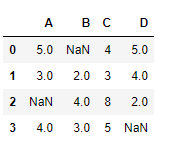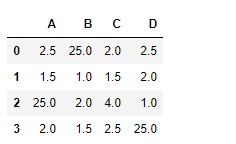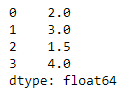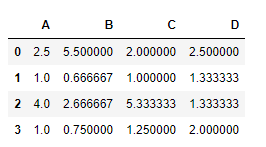Open in App
Not now

# Python | Pandas dataframe.div()

• Difficulty Level : Expert
• Last Updated : 25 Aug, 2021

Python is a great language for doing data analysis, primarily because of the fantastic ecosystem of data-centric python packages. Pandas is one of those packages and makes importing and analyzing data much easier.
Pandas dataframe.div() is used to find the floating division of the dataframe and other element-wise. This function is similar to dataframe/other, but with an additional support to handle missing value in one of the input data.

Syntax: DataFrame.div(other, axis=’columns’, level=None, fill_value=None)
Parameters:
other : Series, DataFrame, or constant
axis : For Series input, axis to match Series index on
fill_value : Fill missing (NaN) values with this value. If both DataFrame locations are missing, the result will be missing
level : Broadcast across a level, matching Index values on the passed MultiIndex level
Returns: result : DataFrame

Example #1: Use div() function to find floating division of dataframe elements with a constant value. Also handle the NaN value present in the dataframe.

## Python3

 `# importing pandas as pd``import` `pandas as pd` `# Creating the dataframe with NaN value``df ``=` `pd.DataFrame({``"A"``:[``5``, ``3``, ``None``, ``4``],``                   ``"B"``:[``None``, ``2``, ``4``, ``3``],``                   ``"C"``:[``4``, ``3``, ``8``, ``5``],``                   ``"D"``:[``5``, ``4``, ``2``, ``None``]})` `# Print the dataframe``df`Now find the division of each dataframe element with 2

## Python3

 `# Find the division with 50 being substituted``# for all the missing values in the dataframe``df.div(``2``, fill_value ``=` `50``)`

Output :The output is a dataframe with cells containing the result of the division of each cell value with 2. All the NaN cells have been filled with 50 before performing the division.

Example #2: Use div() function to find the floating division of a dataframe with a series object over the index axis.

## Python3

 `# importing pandas as pd``import` `pandas as pd` `# Creating the dataframe``df ``=` `pd.DataFrame({``"A"``:[``5``, ``3``, ``6``, ``4``],``                   ``"B"``:[``11``, ``2``, ``4``, ``3``],``                   ``"C"``:[``4``, ``3``, ``8``, ``5``],``                   ``"D"``:[``5``, ``4``, ``2``, ``8``]})` `# Create a series object with no. of elements``# equal to the element along the index axis.` `# Creating a pandas series object``series_object ``=` `pd.Series([``2``, ``3``, ``1.5``, ``4``])` `# Print the series_obejct``series_object`

Output :Note: If the dimension of the index axis of the dataframe and the series object is not same then an error will occur.
Now, find the division of dataframe elements with the series object along the index axis

## Python3

 `# To find the division``df.div(series_object, axis ``=` `0``)`

Output :The output is a dataframe with cells containing the result of the division of the current cell element with the corresponding series object cell.

My Personal Notes arrow_drop_up